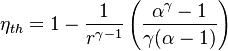# Power Comparison: 5 Parts Air v 800 PSI w/ Diesel

• Automotive
• Hybrid_engine
So the volume of air drawn in will be three times as much for Engine 2. This means that Engine 2 will produce three times as much power.f

#### Hybrid_engine

What is better for power? 5 parts air at 400 psi compression 10 inch bore by 30 inch stroke compared to 800 psi compression with 1 part air with 10 inch bore with 10 inch stroke? Same air fuel ratio in both With diesel? Which will be more power and which will be more efficient? And why?

Compression ratio:
$$r = \left(\frac{P}{P_0}\right)^\frac{1}{1.4}$$
where $P_0$ = 14.7 psi.

Engine 1 ($P$ = 400 psi): $r = 10.59$.
Engine 2 ($P$ = 800 psi): $r = 17.37$.

Diesel cycle thermal efficiency:If we assume $\alpha = 2$ (cut-off ratio) and $\gamma= 1.4$, then:
$$\eta_{th} = 1 - \frac{1.17}{r^{0.4}}$$
Engine 1: $\eta_{th} = 0.544$.
Engine 2: $\eta_{th} = 0.626$.

Engine 2 is more efficient.

Air volume per cycle (at atmospheric pressure):
[Here, I'm not sure what you mean by «5 parts air» and «1 part air»; I'm assuming you mean one engine's rpm is 5X faster than the other one or one has 5 cylinders and the other one has 1 cylinder]
$$V \propto ND^2S$$
and the energy per cycle is:
$$E \propto \eta_{th}V$$
$$\frac{E_1}{E_2} = \frac{\left(\eta_{th}ND^2S\right)_1}{\left(\eta_{th}ND^2S\right)_2} = \frac{0.544 \times 5 \times 10^2 \times 30}{0.626 \times 1 \times 10^2 \times 10} = 13$$
Engine 1 should produce 13 times more power than engine 2.

•Rx7man
Compression ratio:
$$r = \left(\frac{P}{P_0}\right)^\frac{1}{1.4}$$
where $P_0$ = 14.7 psi.

Engine 1 ($P$ = 400 psi): $r = 10.59$.
Engine 2 ($P$ = 800 psi): $r = 17.37$.

Diesel cycle thermal efficiency:If we assume $\alpha = 2$ (cut-off ratio) and $\gamma= 1.4$, then:
$$\eta_{th} = 1 - \frac{1.17}{r^{0.4}}$$
Engine 1: $\eta_{th} = 0.544$.
Engine 2: $\eta_{th} = 0.626$.

Engine 2 is more efficient.

Air volume per cycle (at atmospheric pressure):
[Here, I'm not sure what you mean by «5 parts air» and «1 part air»; I'm assuming you mean one engine's rpm is 5X faster than the other one or one has 5 cylinders and the other one has 1 cylinder]
$$V \propto ND^2S$$
and the energy per cycle is:
$$E \propto \eta_{th}V$$
$$\frac{E_1}{E_2} = \frac{\left(\eta_{th}ND^2S\right)_1}{\left(\eta_{th}ND^2S\right)_2} = \frac{0.544 \times 5 \times 10^2 \times 30}{0.626 \times 1 \times 10^2 \times 10} = 13$$
Engine 1 should produce 13 times more power than engine 2.
By parts of air i mean volume of air.

I still don't understand what you mean with that.. Volume has specific units, so giving us "parts of air" when you also mention bore, stroke, and compression pressure is all a bit confusing.

+1

The volume of air drawn in is usually roughly equal to the displacement of the engine. Engine 2 has three times the displacement of Engine 1.